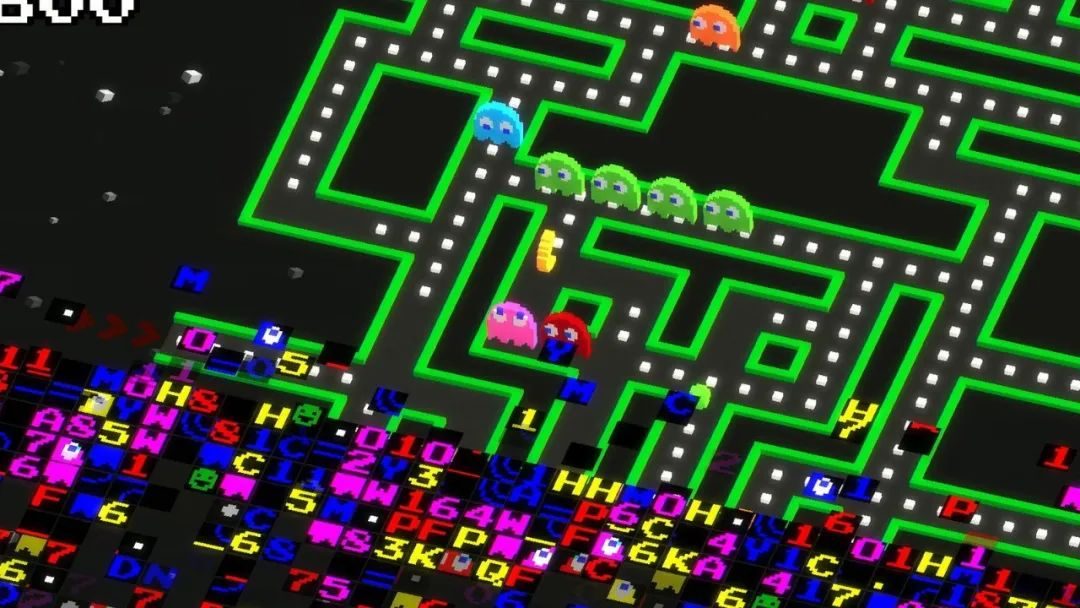# C 语言编程中的 5 个常见错误及对应解决方案

407 篇文章 67 订阅 ¥19.90 ¥99.00https://linux.cn/article-13894-1.html

C 语言有时名声不太好，因为它不像近期的编程语言（比如 Rust）那样具有内存安全性。但是通过额外的代码，一些最常见和严重的 C 语言错误是可以避免的。下文讲解了可能影响应用程序的五个错误以及避免它们的方法：1、未初始化的变量

#include <stdio.h>
#include <stdlib.h>

int
main()
{
int i, j, k;
int numbers;
int *array;

puts("These variables are not initialized:");

printf("  i = %d\n", i);
printf("  j = %d\n", j);
printf("  k = %d\n", k);

puts("This array is not initialized:");

for (i = 0; i < 5; i++) {
printf("  numbers[%d] = %d\n", i, numbers[i]);
}

puts("malloc an array ...");
array = malloc(sizeof(int) * 5);

if (array) {
puts("This malloc'ed array is not initialized:");

for (i = 0; i < 5; i++) {
printf("  array[%d] = %d\n", i, array[i]);
}

free(array);
}

/* done */

puts("Ok");
return 0;
}

These variables are not initialized:
i = 0
j = 0
k = 32766
This array is not initialized:
numbers = 0
numbers = 0
numbers = 4199024
numbers = 0
numbers = 0
malloc an array ...
This malloc'ed array is not initialized:
array = 0
array = 0
array = 0
array = 0
array = 0
Ok

These variables are not initialized:
i = 0
j = 1074
k = 3120
This array is not initialized:
numbers = 3106
numbers = 1224
numbers = 784
numbers = 2926
numbers = 1224
malloc an array ...
This malloc'ed array is not initialized:
array = 3136
array = 3136
array = 14499
array = -5886
array = 219
Ok2、数组越界

C 语言中，数组索引从零开始。这意味着对于长度为 10 的数组，索引是从 0 到 9；长度为 1000 的数组，索引则是从 0 到 999。

#include <stdio.h>
#include <stdlib.h>

int
main()
{
int i;
int numbers;
int *array;

/* test 1 */

puts("This array has five elements (0 to 4)");

/* initalize the array */
for (i = 0; i < 5; i++) {
numbers[i] = i;
}

/* oops, this goes beyond the array bounds: */
for (i = 0; i < 10; i++) {
printf("  numbers[%d] = %d\n", i, numbers[i]);
}

/* test 2 */

puts("malloc an array ...");

array = malloc(sizeof(int) * 5);

if (array) {
puts("This malloc'ed array also has five elements (0 to 4)");

/* initalize the array */
for (i = 0; i < 5; i++) {
array[i] = i;
}

/* oops, this goes beyond the array bounds: */
for (i = 0; i < 10; i++) {
printf("  array[%d] = %d\n", i, array[i]);
}

free(array);
}

/* done */

puts("Ok");
return 0;
}

This array has five elements (0 to 4)
numbers = 0
numbers = 1
numbers = 2
numbers = 3
numbers = 4
numbers = 0
numbers = 4198512
numbers = 0
numbers = 1326609712
numbers = 32764
malloc an array ...
This malloc'ed array also has five elements (0 to 4)
array = 0
array = 1
array = 2
array = 3
array = 4
array = 0
array = 133441
array = 0
array = 0
array = 0
Ok3、字符串溢出

#include <stdio.h>
#include <string.h>

int
main()
{
char name;                       /* Such as "Chicago" */
int var1 = 1, var2 = 2;

/* show initial values */

printf("var1 = %d; var2 = %d\n", var1, var2);

puts("Where do you live?");
gets(name);

/* show ending values */

printf("<%s> is length %d\n", name, strlen(name));
printf("var1 = %d; var2 = %d\n", var1, var2);

/* done */

puts("Ok");
return 0;
}

var1 = 1; var2 = 2
Where do you live?
Raleigh
<Raleigh> is length 7
var1 = 1; var2 = 2
Ok

var1 = 1; var2 = 2
Where do you live?
Llanfairpwllgwyngyllgogerychwyrndrobwllllantysiliogogogoch
<Llanfairpwllgwyngyllgogerychwyrndrobwllllantysiliogogogoch> is length 58
var1 = 2036821625; var2 = 2003266668
Ok
Segmentation fault (core dumped)4、重复释放内存

“分配的内存要手动释放”是良好的 C 语言编程原则之一。程序可以使用 malloc 函数为数组和字符串分配内存，该函数会开辟一块内存，并返回一个指向内存中起始地址的指针。之后，程序可以使用 free 函数释放内存，该函数会使用指针将内存标记为未使用。

#include <stdio.h>
#include <stdlib.h>

int
main()
{
int *array;

puts("malloc an array ...");

array = malloc(sizeof(int) * 5);

if (array) {
puts("malloc succeeded");

puts("Free the array...");
free(array);
}

puts("Free the array...");
free(array);

puts("Ok");
}

malloc an array ...
malloc succeeded
Free the array...
Free the array...
free(): double free detected in tcache 2
Aborted (core dumped)5、使用无效的文件指针

#include <stdio.h>

int
main()
{
FILE *pfile;
int ch;

puts("Open the FILE.TXT file ...");

pfile = fopen("FILE.TXT", "r");

/* you should check if the file pointer is valid, but we skipped that */

puts("Now display the contents of FILE.TXT ...");

while ((ch = fgetc(pfile)) != EOF) {
printf("<%c>", ch);
}

fclose(pfile);

/* done */

puts("Ok");
return 0;
}

Open the FILE.TXT file ...
Now display the contents of FILE.TXT ...
Segmentation fault (core dumped)

via: https://opensource.com/article/21/10/programming-bugs

03-02
05-293853
12-111017
07-31787
08-252525
05-171493
05-181513
05-18561
02-28972
09-175239
02-281万+
05-191547
03-081万+
05-17959
06-181713
10-23793
07-145181
10-20376
07-261900

### “相关推荐”对你有帮助么？

•非常没帮助
•没帮助
•一般
•有帮助
•非常有帮助被折叠的  条评论 为什么被折叠?到【灌水乐园】发言点击重新获取扫码支付1.余额是钱包充值的虚拟货币，按照1:1的比例进行支付金额的抵扣。
2.余额无法直接购买下载，可以购买VIP、C币套餐、付费专栏及课程。余额充值